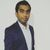Announcements
Sept. 27, 10AM ET Do more with Qlik, Insight Advisor – our intelligent AI-assistant in Qlik Sense: Register
cancel
Showing results for
Did you mean:Anonymous
Not applicable

## Current Date

Hi,

I have to calculate the sales contribution of the customers.

Am using this expression

(sum({\$<DateKey={">=\$(=Date(vFromDate,'YYYYMMDD'))<=\$(=Date(vToDate,'YYYYMMDD'))"}, Year=, Quarter=, Month=, WeekName=, Date=, CustomerCode>} \$(eSales))

- sum({\$<DateKey={">=\$(=Date(vFromDate,'YYYYMMDD'))<=\$(=Date(vToDate,'YYYYMMDD'))"}, Year=, Quarter=, Month=, WeekName=, Date=, CustomerCode>} \$(eRent)))

/

(sum(TOTAL {\$<DateKey={">=\$(=Date(vFromDate,'YYYYMMDD'))<=\$(=Date(vToDate,'YYYYMMDD'))"}, Year=, Quarter=, Month=, WeekName=, Date=>} \$(eSales))

-sum(TOTAL {\$<DateKey={">=\$(=Date(vFromDate,'YYYYMMDD'))<=\$(=Date(vToDate,'YYYYMMDD'))"}, Year=, Quarter=, Month=, WeekName=, Date=>} \$(eRent)))

This is working but i need to show current date's sales contribution without any selection.

.

1 Solution

Accepted SolutionsMVP

May be this

(Sum({\$<DateKey = {"\$(=Date(Today(),'YYYYMMDD'))"}, Year, Quarter, Month, WeekName, Date, CustomerCode>} \$(eSales))

- Sum({\$<DateKey = {"\$(=Date(Today(),'YYYYMMDD'))"}, Year, Quarter, Month, WeekName, Date, CustomerCode>} \$(eRent)))

/

(Sum(TOTAL {\$<DateKey = {"\$(=Date(Today(),'YYYYMMDD'))"}, Year, Quarter, Month, WeekName, Date>} \$(eSales))

-Sum(TOTAL {\$<DateKey = {"\$(=Date(Today(),'YYYYMMDD'))"}, Year, Quarter, Month, WeekName, Date>} \$(eRent)))

12 RepliesMVP

May be this

(Sum({\$<DateKey = {"\$(=Date(Today(),'YYYYMMDD'))"}, Year, Quarter, Month, WeekName, Date, CustomerCode>} \$(eSales))

- Sum({\$<DateKey = {"\$(=Date(Today(),'YYYYMMDD'))"}, Year, Quarter, Month, WeekName, Date, CustomerCode>} \$(eRent)))

/

(Sum(TOTAL {\$<DateKey = {"\$(=Date(Today(),'YYYYMMDD'))"}, Year, Quarter, Month, WeekName, Date>} \$(eSales))

-Sum(TOTAL {\$<DateKey = {"\$(=Date(Today(),'YYYYMMDD'))"}, Year, Quarter, Month, WeekName, Date>} \$(eRent)))Master

What is this field?vToDate

In expression, you can put today's date in filterAnonymous
Not applicable
Author

Thanks you..

How can I create an alert if the sales contribution is greater than 10%MVP

Like an email alert or some in the dashboard itself?Anonymous
Not applicable
Author

An emailMVP

You might be able to use nPrinting... but there might be other ways, but I have not use those so would be difficult to comment about themAnonymous
Not applicable
Author

Ok..Is it possible to show in the dashboard itself like an alert after every reloadMVPAnonymous
Not applicable
Author

Ok..But how can I use this expression as a condition while creating an alert.Community Browser